# Synthesizing Birdsong via Direct Digital Synthesis¶

## Introduction¶

We will be using direct digital synthesis to generate the call of the northern cardinal. Specifically, this adult male northern cardinal recorded by Gerrit Vyn in 2006. This bird was recorded in Texas, but cardinals are also common in Ithaca and throughout the eastern United States. If you pay attention when you're walking through campus, you may hear one singing. The males are a very striking red. You can read more about the cardinal here.

Cardinals have a variety of songs and calls. We will be synthesizing on of its most common songs, which you can hear in the first ten seconds of the recording below:

Here is a screenshot of the spectrogram for the song that we'll be synthesizing. Cardinals and many other songbirds produce almost pure frequency-modulated tones. As can be seen in the spectrogram below, the cardinal sweeps through frequencies from ~2kHz to ~7kHz. We'll assume that the dominant tones (the darkest lines on the spectrogram) are significantly louder than all other frequencies (the lighter lines). We'll only synthesize these loudest frequencies. The generated song sounds quite realistic under this assumption.## Deconstructing the song¶

This song can be decomposed into three sound primitives: a low-frequency swoop at the beginning of each call, a chirp after each swoop which moves rapidly from low frequency to high frequency, and silence which separates each swoop/chirp combination. We will synthesize each of these primitives separately, and then compose them to reconstruct the song.

### Swoop¶

#### Swoop Analysis¶

By pasting the above spectrogram in Keynote or PowerPoint and drawing lines on it, you can determine that the length of the chirp is approximately 130 ms. Since the DAC gathers audio samples at 44kHz, this means that the chirp lasts for $0.130\text{sec} \cdot \frac{44000\text{ samples}}{1\text{ sec}} = 5720\text{ samples}$. We'll approximate the frequency curve by sine wave of the form:

\begin{align} y = k\sin{mx} + b \end{align}

where $y$ is the frequency in Hz, and $x$ is the number of audio samples since the chirp began. Since the swoop starts and ends at 1.74kHz and peaks at 2kHz, we can setup the following system of equations to solve for the unknown parameters $k$, $b$, and $m$:

\begin{align} 1740 &= k\sin{m\cdot 0} + b\\ 1740 &= k\sin{m\cdot 5720} + b\\ 2000 &= k\sin{m\cdot 2860} + b \end{align}

From which we can find $b=1740$, $k = -260$, $m = \frac{-\pi}{5720}$. So, the equation is given by:

\begin{align} y = -260 \sin{\left(-\frac{\pi}{5720} \cdot x\right)} + 1740 \end{align}

Plot the simulated swoop of frequencies:

In :
plt.plot(-260*numpy.sin(-numpy.pi/5720*numpy.arange(5720)) + 1740)
plt.xlabel('Audio samples'); plt.ylabel('Hz'); plt.title('Swoop frequencies')
plt.show()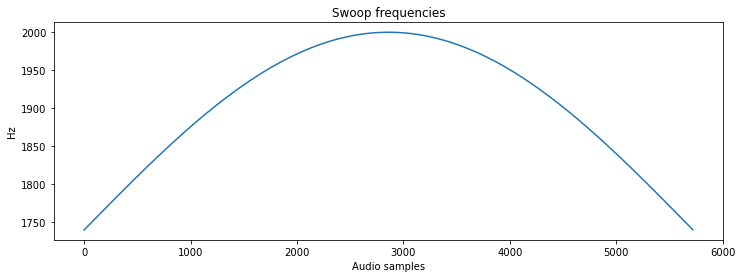#### Swoop Simulation¶

Some DDS parameters, including the sample rate (44kHz), a 256-entry sine table, and the constant $2^{32}$:

In :
Fs = 44000 #audio sample rate
sintable = numpy.sin(numpy.linspace(0, 2*numpy.pi, 256))# sine table for DDS
two32 = 2**32 #2^32


And now we can synthesize audio samples.

In :
swoop = list(numpy.zeros(5720)) # a 5720-length array (130ms @ 44kHz) that will hold swoop audio samples
DDS_phase = 0 # current phase
for i in range(len(swoop)):
frequency = -260.*numpy.sin((-numpy.pi/5720)*i) + 1740 # calculate frequency
DDS_increment = frequency*two32/Fs # update DDS increment
DDS_phase += DDS_increment # update DDS phase by increment
DDS_phase = DDS_phase % (two32 - 1) # need to simulate overflow in python, not necessary in C
swoop[i] = sintable[int(DDS_phase/(2**24))] # can just shift in C


In order to avoid non-natural clicks, we must ramp the amplitude smoothly from 0 to its max amplitude, and then ramp it down. We'll do this by multiplying the chirp by the linear ramp function shown below:

In :
# Amplitude modulate with a linear envelope to avoid clicks
amplitudes = list(numpy.ones(len(swoop)))
amplitudes[0:1000] = list(numpy.linspace(0,1,len(amplitudes[0:1000])))
amplitudes[-1000:] = list(numpy.linspace(0,1,len(amplitudes[-1000:]))[::-1])
amplitudes = numpy.array(amplitudes)
plt.plot(amplitudes);plt.title('Amplitude envelope');plt.show()

# Finish with the swoop
swoop = swoop*amplitudes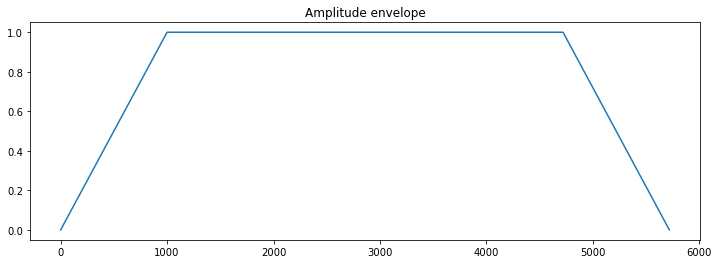Here is what the swoop looks like:

In :
plt.plot(swoop);plt.title('Amplitude-modulated swoop');plt.show()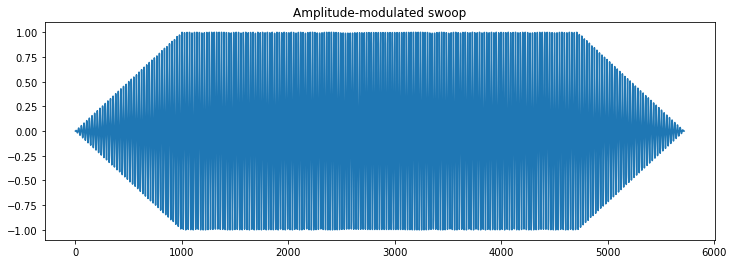And here is what it sounds like:

In :
Audio(swoop, rate=48000)

Out:

### Chirp¶

#### Chirp Analysis¶

By pasting the above spectrogram in Keynote and drawing lines on it, you can determine that the length of the chirp is also approximately 130 ms (5720 samples). We'll approximate the frequency curve by a quadratic equation of the form:

\begin{align} y = kx^2 + b \end{align}

where $y$ is the frequency in Hz, and $x$ is the number of audio samples since the chirp began. Since the chirp starts at 2kHz and ends at 7kHz, we can setup the following system of equations to solve for the unknown parameters $k$ and $b$:

\begin{align} 2000 &= k(0^2) + b\\ 7000&= k(5720^2) + b \end{align}

This is two equations with two unknowns. Solving, we find that $b=2000$ and $k\approx 1.53 \times 10^{-4}$. So the quadratic equation for the chirp is:

\begin{align} y &= \left(1.53\times 10^{-4}\right)x^2 + 2000 && \text{for $x\in[0, 5720]$} \end{align}

Plot the simulated chirp frequency sweep:

In :
plt.plot(1.53e-4 * numpy.arange(5720)**2. + 2000);plt.title('Chirp frequencies');plt.show()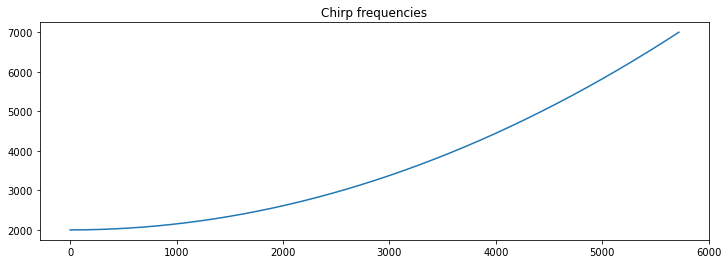#### Chirp Simulation¶

And now we can synthesize audio samples.

In :
chirp = list(numpy.zeros(5720)) # a 5720-length array (130ms @ 44kHz) that will hold chirp audio samples
DDS_phase = 0 # current phase
for i in range(len(chirp)):
frequency = (1.53e-4)*(i**2.) + 2000 # update DDS frequency
DDS_increment = frequency*two32/Fs # update DDS increment
DDS_phase += DDS_increment # update DDS phase
DDS_phase = DDS_phase % (two32 - 1) # need to simulate overflow in python, not necessary in C
chirp[i] = sintable[int(DDS_phase/(2**24))] # can just shift in C


In order to avoid non-natural clicks, we must ramp the amplitude smoothly from 0 to its max amplitude, and then ramp it down. We'll do this by multiplying the chirp by the linear ramp function shown below:

In :
# Amplitude modulate with a linear envelope to avoid clicks
amplitudes = list(numpy.ones(len(chirp)))
amplitudes[0:1000] = list(numpy.linspace(0,1,len(amplitudes[0:1000])))
amplitudes[-1000:] = list(numpy.linspace(0,1,len(amplitudes[-1000:]))[::-1])
amplitudes = numpy.array(amplitudes)

# Finish with the chirp
chirp = chirp*amplitudes


The entire amplitude-modulated chirp looks like this:

In :
plt.plot(chirp);plt.title('Amplitude-modulated chirp');plt.show()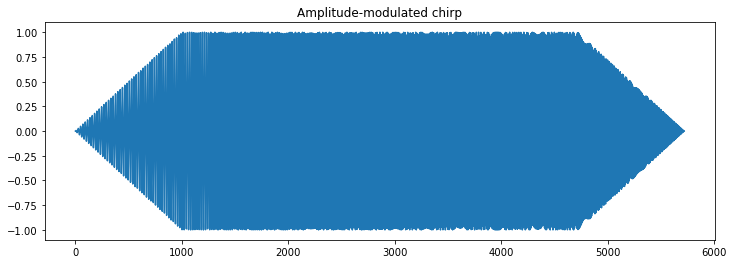And it sounds like this:

In :
Audio(chirp, rate=44000)

Out:

### Silence¶

The amount of time between swoop/chirps is also (approximately) 130ms or 5720 cycles.

In :
silence = numpy.zeros(5720)


### Assembling the song¶

We assemble the song by playing the swoop/chirp/silence in succession:

In :
song = []
for i in range(5):
song.extend(list(swoop))
song.extend(list(chirp))
song.extend(list(silence))
song.extend(list(swoop))
song.extend(list(chirp))
song.extend(list(silence))
song = numpy.array(song)

plt.plot(song);plt.title('Full song');plt.show()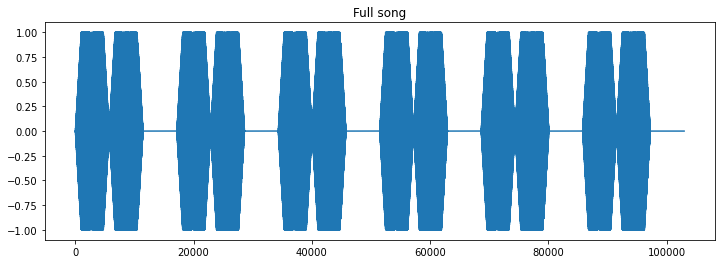Listen to it:

In :
Audio(song, rate=44000)

Out:

And view the spectrogram of the Python-generated song:

In :
f, t, Sxx = signal.spectrogram(song, Fs)
plt.pcolormesh(t, f, Sxx,  shading='gouraud')
plt.ylabel('Hz'); plt.xlabel('Time (sec)')
plt.title('Spectrogram of Python-generated birdsong')
plt.ylim([0,10000])
plt.show()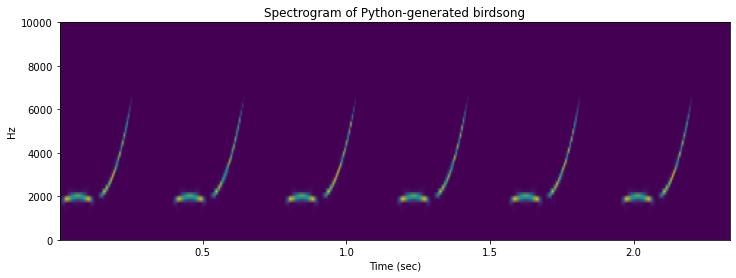In :
import numpy
import matplotlib.pyplot as plt
from IPython.display import Audio
from IPython.display import Image
from scipy import signal
from scipy.fft import fftshift
from scipy.io import wavfile
plt.rcParams['figure.figsize'] = [12, 4]
from IPython.core.display import HTML
HTML("""
<style>
.output_png {
display: table-cell;
text-align: center;
vertical-align: middle;
}
</style>
""")

Out: